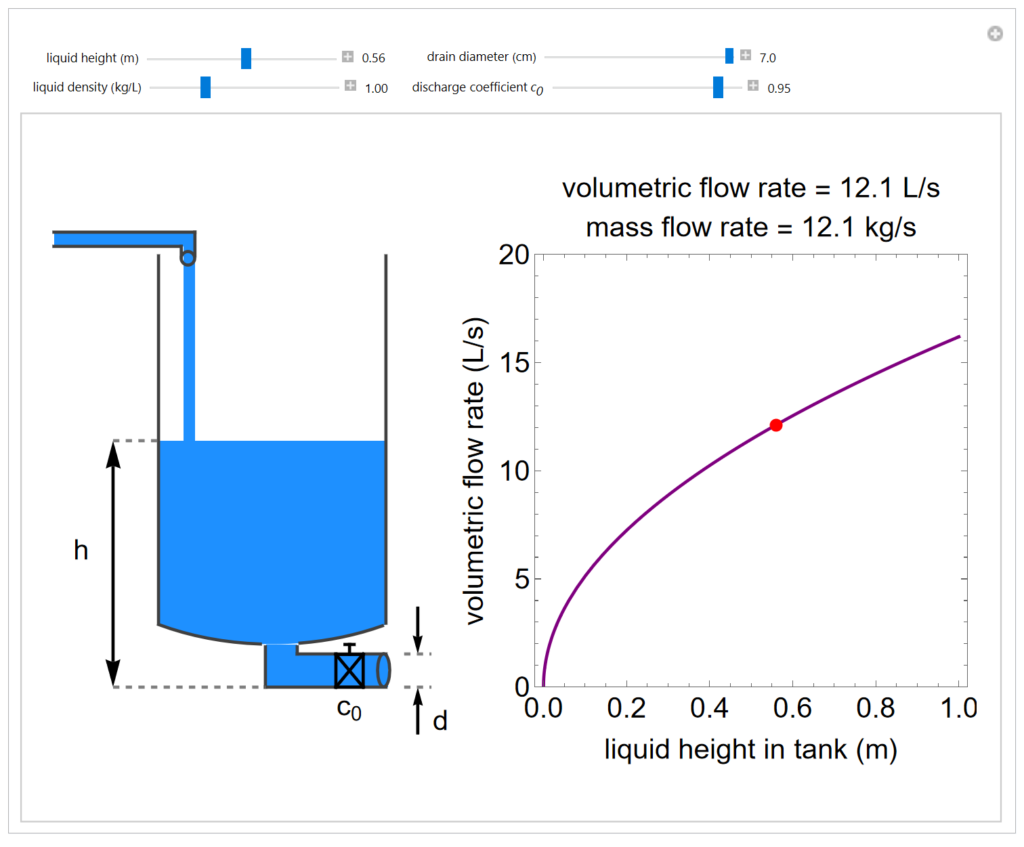LearnChemE

##### Steady-state Flow from a Tank##### Description

This simulation calculates and displays the volumetric and mass flow rates of a liquid maintained at a constant height in a tank as a function of the liquid’s height $$h$$ and density $$\rho$$, the drain pipe’s diameter $$d$$ and the discharge (orifice) coefficient $$c_{0}$$. It also plots the volumetric flow rate curve as a function of the liquid’s height for the chosen drain diameter and discharge coefficient values and displays a schematic diagram of the system. The discharge (orifice) coefficient $$c_{0}$$ characterizes the relationship between actual flow and ideal flow through an orifice. An orifice with discharge coefficient of 1.0 behaves as if pressure drop across the orifice ($$\Delta p$$) is zero. An orifice with a discharge coefficient near zero restricts flow significantly, even at large pipe diameters.

This simulation runs on desktop using the free Wolfram Player. Download the Wolfram Player here.